机器学习入门“Hello, World”

1. 准备工作if(speed<4){
status=WALKING;
}if(speed<4){
status=WALKING;
} else {
status=RUNNING;
}if(speed<4){
status=WALKING;
} else if(speed<12){
status=RUNNING;
} else {
status=BIKING;
}// Now what?

前提条件

• 扎实的 Python 知识
• 基本编程技能

• 机器学习的基础知识

• 您的首个机器学习模型

2. 什么是机器学习？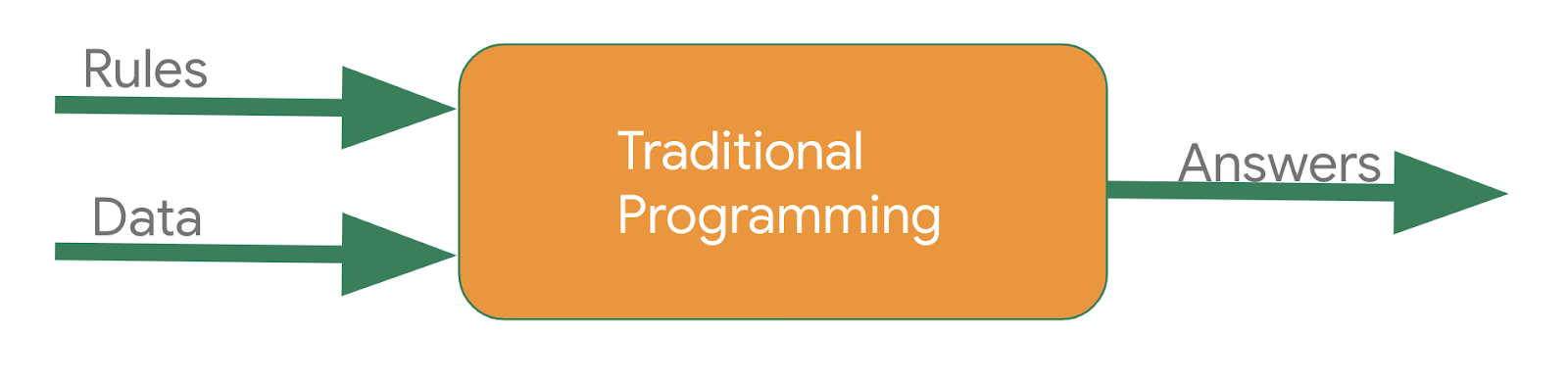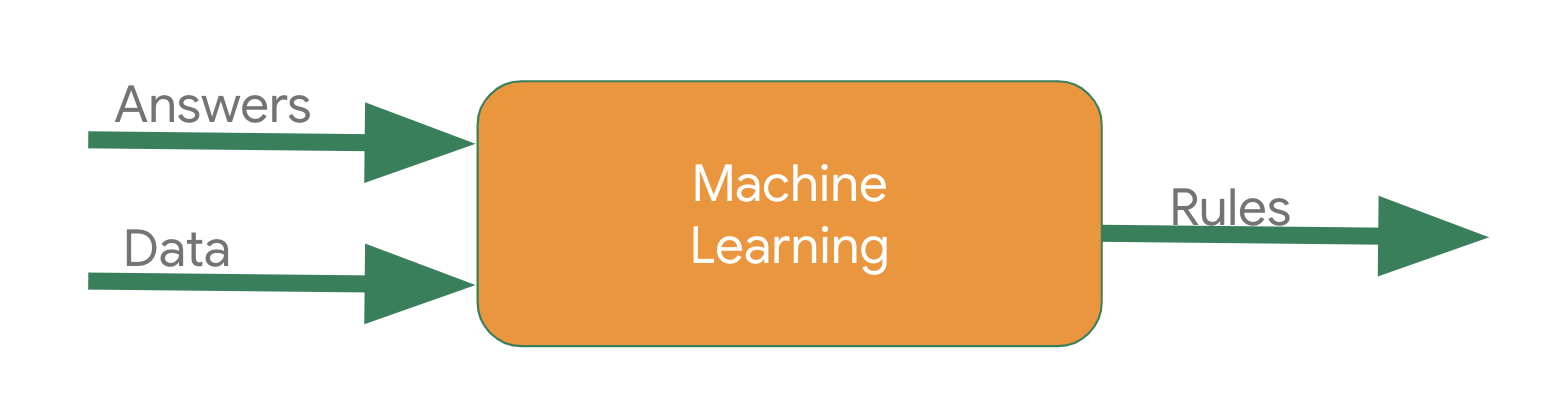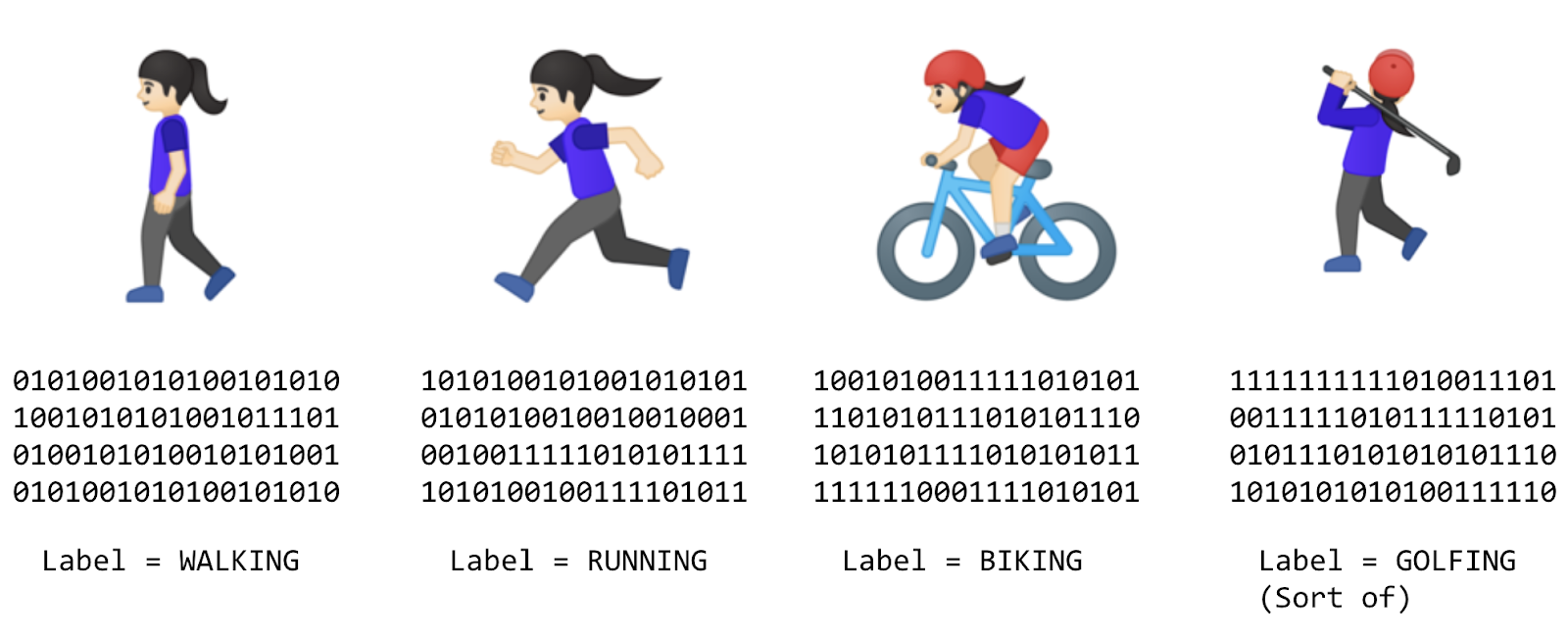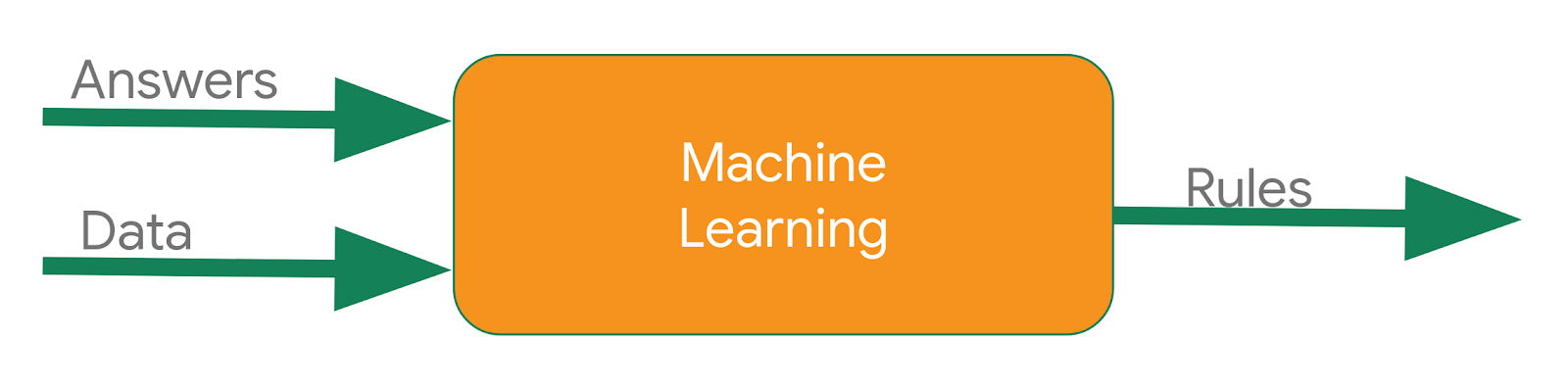3. 创建您的首个机器学习模型

 X: -1 0 1 2 3 4 Y: -2 1 4 7 10 13

导入数据

import tensorflow as tf
import numpy as np
from tensorflow import keras

定义并编译神经网络

model = tf.keras.Sequential([keras.layers.Dense(units=1, input_shape=)])

model.compile(optimizer='sgd', loss='mean_squared_error')

提供数据

xs = np.array([-1.0, 0.0, 1.0, 2.0, 3.0, 4.0], dtype=float)
ys = np.array([-2.0, 1.0, 4.0, 7.0, 10.0, 13.0], dtype=float)

4. 训练神经网络

model.fit(xs, ys, epochs=500)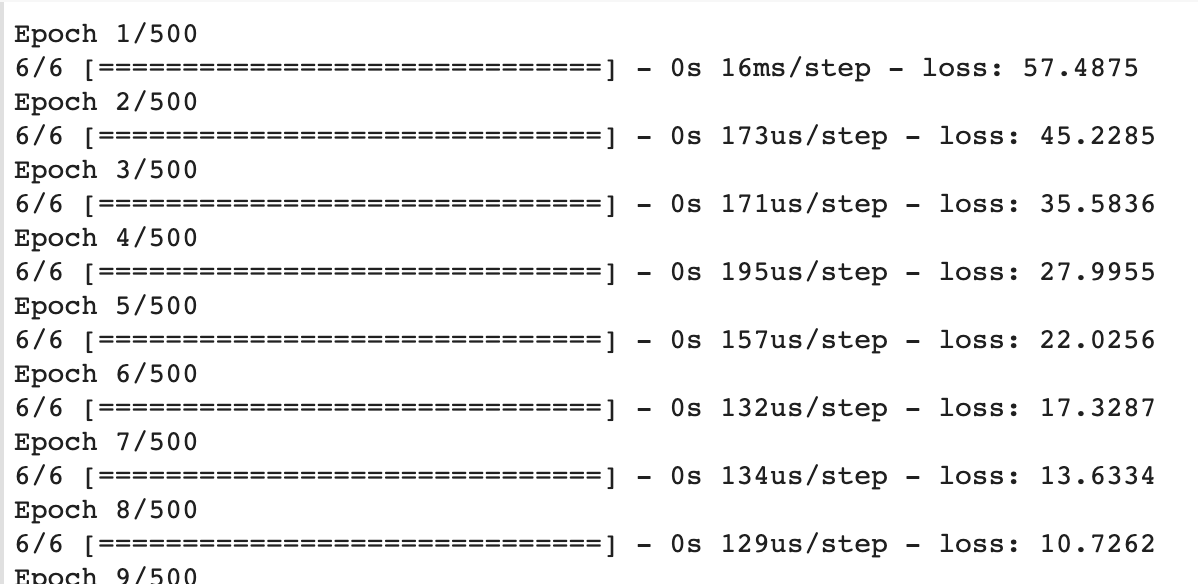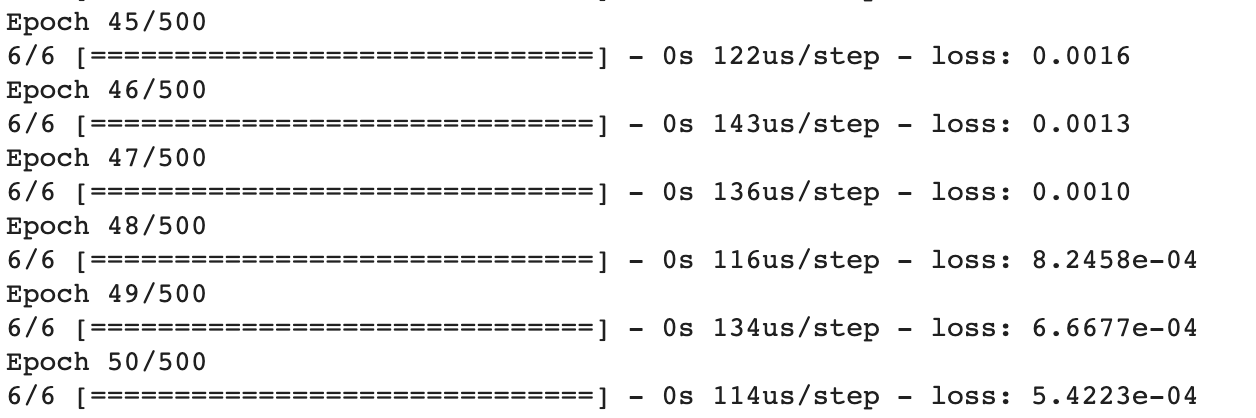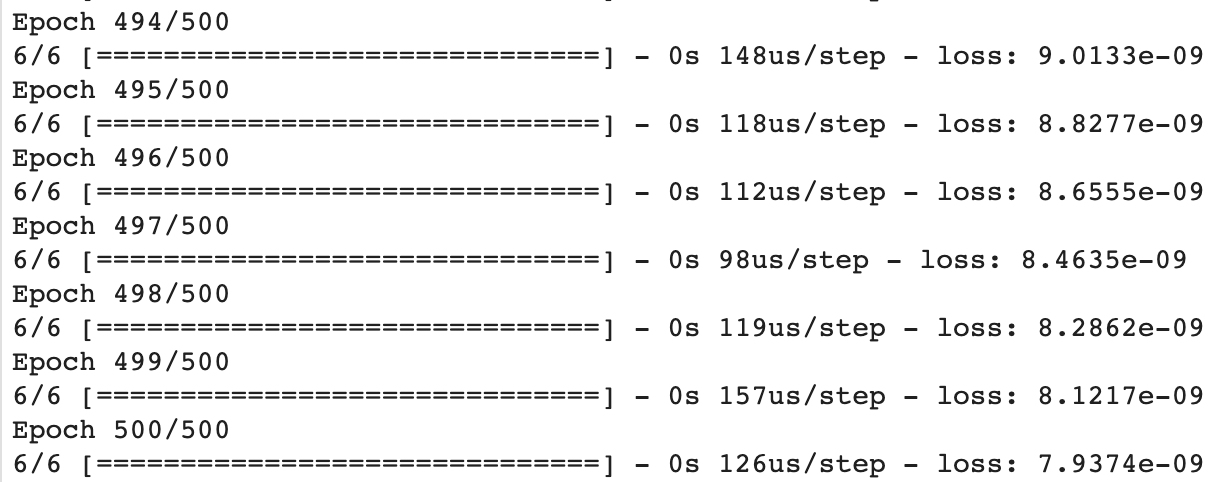5. 使用模型

print(model.predict([10.0]))

[]
[]